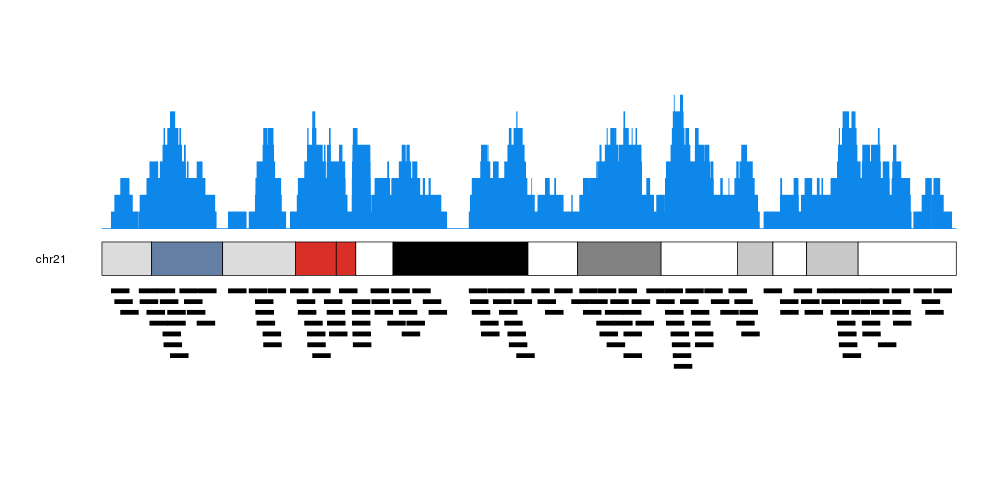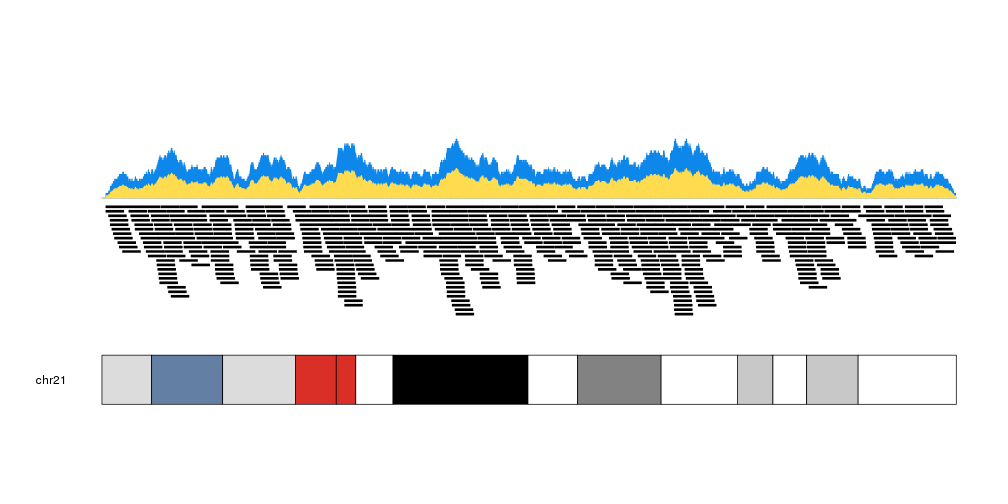Plotting the per base coverage of genomic features

The kpPlotCoverage function is similar to kpPlotDensity but instead of plotting the number of features overalpping a certain genomic window, it plots the actual number of features overlapping every single base of the genome. Conceptually, it is equivalent to kpPlotDensity with window.size set to 1 but much faster, since internally it uses the coverage method from GenomicRanges.

As an example, we’ll create ten thousand random regions of one megabase of mean length using createRandomRegions from package regioneR. We set non.overlapping to FALSE to allow for overlapping between random regions.

library(karyoploteR)
regions <- createRandomRegions(nregions=10000, length.mean = 1e6, mask=NA, non.overlapping=FALSE)
kp <- plotKaryotype()
kpPlotCoverage(kp, data=regions)The actual representation of the data is also different than that of kpPlotDensity: kpPlotCoverage uses kpBars to create the plot, giving it a histogram-like appearance.

We can plot the actual regions in data panel 2 to see how they relate

kp <- plotKaryotype(plot.type=2, chromosomes = "chr21")
kpPlotCoverage(kp, data=regions)
kpPlotRegions(kp, data=regions, data.panel=2)It is possible to customize the appearance of the coverage plot using the same parameters used for kpRect.

more.regions <- createRandomRegions(nregions=40000, length.mean = 1e6, mask=NA, non.overlapping = FALSE)
kp <- plotKaryotype(plot.type=1, chromosomes = "chr21")
kpPlotCoverage(kp, data=more.regions, r0=0.7, r1=1, col="#0e87eb")
kpPlotCoverage(kp, data=more.regions, r0=0.7, r1=0.85, col="#ffdb50")
kpPlotRegions(kp, data=more.regions, r0=0.65, r1=0)